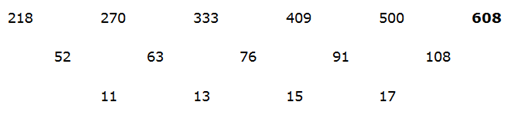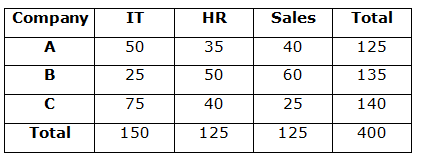# SBI Clerk Prelims 2021 Quantitative Aptitude Questions (Day-24)

Dear Aspirants, Our IBPS Guide team is providing new series of Quantitative Aptitude Questions for SBI Clerk Prelims 2020 so the aspirants can practice it on a daily basis. These questions are framed by our skilled experts after understanding your needs thoroughly. Aspirants can practice these new series questions daily to familiarize with the exact exam pattern and make your preparation effective.

Start Quiz

Missing number series

Directions (01-05): What value should come in place of (?) in the following number series?

1) 73, 37, 38, 58.5, 119,?

A.298

B.302

C.300

D.294

E.306

2) 52, 274, 404, 472, 502, ?

A.517

B.522

C.512

D.532

E.412

3) 126, 64, 72, 171, ? , 6109

A.748

B.648

C.948

D.848

E.628

4) 218, 270, 333, 409, 500, ?

A.722

B.686

C.712

D.608

E.618

5) 1290, 645, 430,? , 258, 215

A.317.5

B.322.5

C.215

D.332.5

E.324.25

Caselet

Directions (06-10): Study the following information carefully and answer the questions given below.

There are three companies A, B and C have the three different departments – IT, HR and Sales. The total number of employees in all the companies together is 400. Total number of employees in company B is 135. The number of IT employees in company B is 25 which is equal to the number of Sales employees in company C.  The ratio of the IT employees in company A to C is 2: 3 and the number of HR employees in company B is 50. The number of IT employees in company C is sum of the HR employees of company A and C. The number of Sales employees in company B is 25 more than the number of HR employees in company A. The total number of IT employees in all the companies together is 150.

6) What is the ratio of the number of HR employees in company A to the number of Sales employees in company B?

A.2: 3

B.5: 9

C.8: 13

D.7: 12

E.None of these

7) The number of Sales employees in company A and C together is approximately what percent of the total number of IT employees in all the companies together?

A.40%

B.43%

C.47%

D.45%

E.49%

8) What is the total number of HR employees in all the companies together?

A.130

B.120

C.125

D.115

E.None of these

9) What is the difference between the total number of employees in company A and C?

A.20

B.25

C.15

D.10

E.None of these

10) What is the ratio of the sum of the number of IT employees in company C and the number of HR employees in company B together to the total number of Sales employees in all the companies together?

A.1: 1

B.2: 1

C.2: 3

D.3: 4

E.None of these

73 x 0.5 + 0.5 = 37

37 x 1 + 1 = 38

38 x 1.5 + 1.5 = 58.5

58.5 x 2 + 2 = 119

119 x 2.5 + 2.5 = 300

52 + 6+ 6= 274

274 + 53 + 5 = 404

404 + 43 + 4 = 472

472 + 33 + 3 = 502

502 + 2+ 2 = 512

126 x 0.5 + 1= 64

64 x 1 + 23 = 72

72 x 2 + 33 = 171

171 x 4 + 43 = 748

748 x 8 + 53 = 61091290 x ½ = 645

645 x 2/3 = 430

430 x ¾ = 322.5

322.5 x 4/5 = 258

258 x 5/6 = 215

Directions (6-10):

Total number of employees = 400

Total number of IT employees = 150

Number of IT employees in company B = 25

Number of IT employees in company A = 2/5 * 125 = 50

Number of IT employees in company C = 3/5 * 125 = 75

Number of HR employees in company B = 50

Number of Sales employees in company B = 135 – 50 – 25 = 60

Number of Sales employees in company C = 25

Number of HR employees in A = 60 – 25 = 35

Number of HR employees in C = 75 – 35 = 40

Total number of HR employees = 50 + 35 + 40 = 125

Number of Sales employees in company A = 400 – 150 – 125 – 25 – 60 = 40

Total number of Sales employees = 40 + 60 + 25 = 125

Total number of employees in company A = 50 + 35 + 40 = 125

Total number of employees in company B = 135

Total number of employees in company C = 75 + 25 + 40 = 140Required ratio = 35: 60

= 7: 12

Required % = (40 + 25)/150 * 100 = 43.33%

HR employees = (35 + 50 + 40) = 125

Difference = 140 – 125 = 15

Required ratio = (75 + 50): (40 + 60 + 25)

= 125: 125

= 1: 1

 Check Here to View SBI Clerk Prelims 2021 Quantitative Aptitude Questions Day – 23 Day – 22 Day – 21